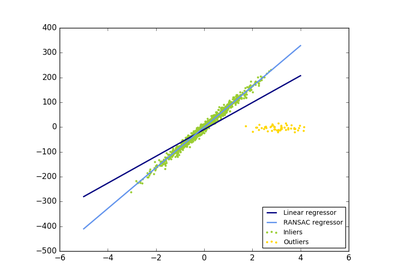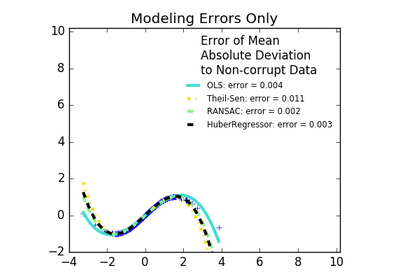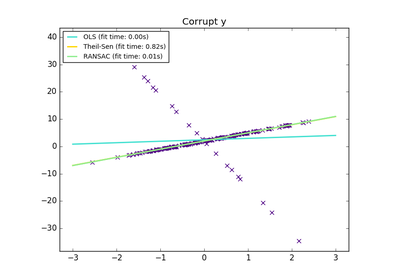# `sklearn.linear_model`.RANSACRegressor¶

class `sklearn.linear_model.``RANSACRegressor`(base_estimator=None, min_samples=None, residual_threshold=None, is_data_valid=None, is_model_valid=None, max_trials=100, stop_n_inliers=inf, stop_score=inf, stop_probability=0.99, residual_metric=None, loss='absolute_loss', random_state=None)[source]

RANSAC (RANdom SAmple Consensus) algorithm.

RANSAC is an iterative algorithm for the robust estimation of parameters from a subset of inliers from the complete data set. More information can be found in the general documentation of linear models.

A detailed description of the algorithm can be found in the documentation of the `linear_model` sub-package.

Read more in the User Guide.

Parameters: base_estimator : object, optional Base estimator object which implements the following methods: fit(X, y): Fit model to given training data and target values. score(X, y): Returns the mean accuracy on the given test data, which is used for the stop criterion defined by stop_score. Additionally, the score is used to decide which of two equally large consensus sets is chosen as the better one. If base_estimator is None, then `base_estimator=sklearn.linear_model.LinearRegression()` is used for target values of dtype float. Note that the current implementation only supports regression estimators. min_samples : int (>= 1) or float ([0, 1]), optional Minimum number of samples chosen randomly from original data. Treated as an absolute number of samples for min_samples >= 1, treated as a relative number ceil(min_samples * X.shape) for min_samples < 1. This is typically chosen as the minimal number of samples necessary to estimate the given base_estimator. By default a `sklearn.linear_model.LinearRegression()` estimator is assumed and min_samples is chosen as `X.shape + 1`. residual_threshold : float, optional Maximum residual for a data sample to be classified as an inlier. By default the threshold is chosen as the MAD (median absolute deviation) of the target values y. is_data_valid : callable, optional This function is called with the randomly selected data before the model is fitted to it: is_data_valid(X, y). If its return value is False the current randomly chosen sub-sample is skipped. is_model_valid : callable, optional This function is called with the estimated model and the randomly selected data: is_model_valid(model, X, y). If its return value is False the current randomly chosen sub-sample is skipped. Rejecting samples with this function is computationally costlier than with is_data_valid. is_model_valid should therefore only be used if the estimated model is needed for making the rejection decision. max_trials : int, optional Maximum number of iterations for random sample selection. stop_n_inliers : int, optional Stop iteration if at least this number of inliers are found. stop_score : float, optional Stop iteration if score is greater equal than this threshold. stop_probability : float in range [0, 1], optional RANSAC iteration stops if at least one outlier-free set of the training data is sampled in RANSAC. This requires to generate at least N samples (iterations): ```N >= log(1 - probability) / log(1 - e**m) ``` where the probability (confidence) is typically set to high value such as 0.99 (the default) and e is the current fraction of inliers w.r.t. the total number of samples. residual_metric : callable, optional Metric to reduce the dimensionality of the residuals to 1 for multi-dimensional target values `y.shape > 1`. By default the sum of absolute differences is used: ```lambda dy: np.sum(np.abs(dy), axis=1) ``` NOTE: residual_metric is deprecated from 0.18 and will be removed in 0.20 Use `loss` instead. loss : string, callable, optional, default “absolute_loss” String inputs, “absolute_loss” and “squared_loss” are supported which find the absolute loss and squared loss per sample respectively. If `loss` is a callable, then it should be a function that takes two arrays as inputs, the true and predicted value and returns a 1-D array with the ``i``th value of the array corresponding to the loss on X[i]. If the loss on a sample is greater than the `residual_threshold`, then this sample is classified as an outlier. random_state : integer or numpy.RandomState, optional The generator used to initialize the centers. If an integer is given, it fixes the seed. Defaults to the global numpy random number generator. estimator_ : object Best fitted model (copy of the base_estimator object). n_trials_ : int Number of random selection trials until one of the stop criteria is met. It is always `<= max_trials`. inlier_mask_ : bool array of shape [n_samples] Boolean mask of inliers classified as `True`.

References

Methods

 `fit`(X, y[, sample_weight]) Fit estimator using RANSAC algorithm. `get_params`([deep]) Get parameters for this estimator. `predict`(X) Predict using the estimated model. `score`(X, y) Returns the score of the prediction. `set_params`(\*\*params) Set the parameters of this estimator.
`__init__`(base_estimator=None, min_samples=None, residual_threshold=None, is_data_valid=None, is_model_valid=None, max_trials=100, stop_n_inliers=inf, stop_score=inf, stop_probability=0.99, residual_metric=None, loss='absolute_loss', random_state=None)[source]
`fit`(X, y, sample_weight=None)[source]

Fit estimator using RANSAC algorithm.

Parameters: X : array-like or sparse matrix, shape [n_samples, n_features] Training data. y : array-like, shape = [n_samples] or [n_samples, n_targets] Target values. sample_weight : array-like, shape = [n_samples] Individual weights for each sample raises error if sample_weight is passed and base_estimator fit method does not support it. ValueError : If no valid consensus set could be found. This occurs if is_data_valid and is_model_valid return False for all max_trials randomly chosen sub-samples.
`get_params`(deep=True)[source]

Get parameters for this estimator.

Parameters: deep : boolean, optional If True, will return the parameters for this estimator and contained subobjects that are estimators. params : mapping of string to any Parameter names mapped to their values.
`predict`(X)[source]

Predict using the estimated model.

This is a wrapper for estimator_.predict(X).

Parameters: X : numpy array of shape [n_samples, n_features] y : array, shape = [n_samples] or [n_samples, n_targets] Returns predicted values.
`score`(X, y)[source]

Returns the score of the prediction.

This is a wrapper for estimator_.score(X, y).

Parameters: X : numpy array or sparse matrix of shape [n_samples, n_features] Training data. y : array, shape = [n_samples] or [n_samples, n_targets] Target values. z : float Score of the prediction.
`set_params`(**params)[source]

Set the parameters of this estimator.

The method works on simple estimators as well as on nested objects (such as pipelines). The latter have parameters of the form `<component>__<parameter>` so that it’s possible to update each component of a nested object.

Returns: self :

## Examples using `sklearn.linear_model.RANSACRegressor`¶Robust linear model estimation using RANSACRobust linear estimator fittingTheil-Sen Regression